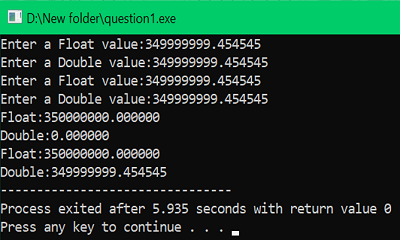# scanf() need '%lf' for doubles, when printf() is okay with just '%f'

Learn, why scanf() function needs "%lf" for doubles but printf() function is okay with "%f" in C programming language?
Submitted by Shubh Pachori, on July 07, 2022

As we know that in C language to store the value of a double variable we use %lf with the scanf() function and %f for the float values. Other than in the printf() function, %f can we use for both the float and double values it is not an ideal way to display but there is no issue with using this with the printf() function? The range of double is about 2.3E-308 to 1.7E+308 and its size is 8 bytes in double we can store values up to 15 digits after the decimal but in float, its size is 4 bytes that is half of the double, and its range is 1.2E-38 to 3.4E+38 and it can store only 6 digits after the decimal.

So, if we use %f instead of %lf for a double variable at the time of storing with the help of the scanf() function it will only store 6 values after the decimal but the %lf can store values up to 15 values after the decimal. So, when we use double we have to store much bigger values than that can be stored in the float variable so if we use %f rather than the %lf for the double variable then it will only store the values which can be stored in float so there is no use of declaring a variable in double.

If we use %f with a double variable then the declared double variable will work as a float with the memory storage of double than the float. i.e; The memory size of a float is 4 bytes and the size of a double is 8 bytes. But in the printf() function we can use the %f for the displaying of the result of a double variable but it is also not the right way to write a code because %f will not display the exact value which is stored in the double variable but it will mess up the value but if we use %lf it will display the exact value which is stored in the double variable. Hence, it is necessary to use %lf instead of %f with a double variable for the exact and smooth result displaying.

Example:

In the below code we have used both %lf and %f to store and print doubles and float values and we can see that there is a slight difference in the values which are stored or printed using %lf and %f.

```#include <stdio.h>

int main()
{
//declaring two float variables
float f1, f2;
// declaring two double variables
double d1, d2;

printf("Enter a Float value:");
scanf("%f", &f1);
printf("Enter a Double value:");
scanf("%f", &d1);

printf("Enter a Float value:");
scanf("%f", &f2);
printf("Enter a Double value:");
scanf("%lf", &d2);

printf("Float:%f\nDouble:%f", f1, d1);
printf("\nFloat:%f\nDouble:%lf", f2, d2);

return 0;
}
```

Output: